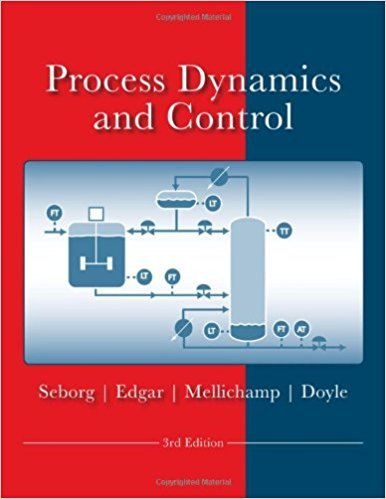×
Get Full Access to Process Dynamics And Control - 3 Edition - Chapter 4 - Problem 4.6
Get Full Access to Process Dynamics And Control - 3 Edition - Chapter 4 - Problem 4.6

×

# A stirred-tank blending system can be described by aISBN: 9780470128671 148

## Solution for problem 4.6 Chapter 4

Process Dynamics and Control | 3rd Edition

• Textbook Solutions
• 2901 Step-by-step solutions solved by professors and subject experts
• Get 24/7 help from StudySoup virtual teaching assistantsProcess Dynamics and Control | 3rd Edition

4 5 1 413 Reviews
27
4
Problem 4.6

A stirred-tank blending system can be described by a firstorder transfer function between the exit composition x and the inlet composition x1 (both are mass fractions of solute):

Step-by-Step Solution:

Problem 4.6A stirred-tank blending system can be described by a first order transfer function between theexit composition x and the inlet composition x1 (both are mass fractions of solute): Step-by-step solution Step 1 of 9 ^(a)The first-order transfer function of the stirred-tank blending system is, X(s) = K ………(1) X is) s + 1Where ,K is dimensionless. is the time constant.Substitute 0.6 for K and 10 for in Equation (1). X(s) = 0.6 ………(2) X is) 10s + 1

Step 2 of 9

Step 3 of 9

##### ISBN: 9780470128671

Process Dynamics and Control was written by and is associated to the ISBN: 9780470128671. Since the solution to 4.6 from 4 chapter was answered, more than 1077 students have viewed the full step-by-step answer. This full solution covers the following key subjects: composition, function, Blending, described, exit. This expansive textbook survival guide covers 24 chapters, and 420 solutions. The full step-by-step solution to problem: 4.6 from chapter: 4 was answered by , our top Engineering and Tech solution expert on 11/15/17, 04:03PM. This textbook survival guide was created for the textbook: Process Dynamics and Control, edition: 3. The answer to “A stirred-tank blending system can be described by a firstorder transfer function between the exit composition x and the inlet composition x1 (both are mass fractions of solute):” is broken down into a number of easy to follow steps, and 28 words.

Unlock Textbook Solution